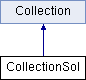ParM  parm A molecular dynamics library
CollectionSol Class Reference

A Collection with a "solvent", using the Langevin equation. More...

#include <collection.hpp>

Inheritance diagram for CollectionSol:## Public Member Functions

CollectionSol (sptr< Box > box, sptr< AtomGroup > atoms, const flt dt, const flt damping, const flt desired_temperature, vector< sptr< Interaction > > interactions=vector< sptr< Interaction > >(), vector< sptr< StateTracker > > trackers=vector< sptr< StateTracker > >(), vector< sptr< Constraint > > constraints=vector< sptr< Constraint > >())

void change_temperature (const flt damp, const flt desired_temperature)
Change the desired damping coefficient $$\xi$$ or temperature $$T$$. More...

void change_force (const flt damp, const flt fmag, const flt desired_temperature)

void set_dt (const flt newdt)

void timestep ()
Take one step forward in time. More...Public Member Functions inherited from Collection
Collection (sptr< Box > box, sptr< AtomGroup > atoms, vector< sptr< Interaction > > interactions=vector< sptr< Interaction > >(), vector< sptr< StateTracker > > trackers=vector< sptr< StateTracker > >(), vector< sptr< Constraint > > constraints=vector< sptr< Constraint > >(), bool should_initialize=true)

virtual void initialize ()

virtual void set_forces (bool constraints_and_a=true)
Set forces. More...

flt degrees_of_freedom ()
Total degrees of freedom. More...

flt potential_energy ()

flt energy ()
Total energy, including both potential and kinetic_energy. More...

virtual flt temp (bool minuscomv=true)

virtual flt kinetic_energy ()

virtual flt virial ()

virtual flt pressure ()
Returns (1/d V) Σ ri dot fi where d is number of dimensions note that Interaction->pressure just returns Σ ri dot fi. More...

sptr< Boxget_box ()

Vec com ()

Vec com_velocity ()

Vec angular_momentum (const Vec &loc)
Shortcut to AtomGroup method of the same name. More...

Vec angular_momentum ()
Shortcut to AtomGroup method of the same name. More...

virtual ~Collection ()

void reset_com_velocity ()
Shortcut to AtomGroup method of the same name. More...

void reset_L ()
Shortcut to AtomGroup method of the same name. More...

void scale_velocities (flt scaleby)
Scale all velocities by a factor. More...

void scale_velocities_to_temp (flt T, bool minuscomv=true)
Scale all velocities to get to a specific temperature. More...

void scale_velocities_to_energy (flt E)
Scale all velocities to get to a specific total energy. More...

void add_interaction (sptr< Interaction > inter)

void add_tracker (sptr< StateTracker > track)

void add_constraint (sptr< Constraint > c)

void add (sptr< Interaction > a)

void add (sptr< StateTracker > a)

void add (sptr< Constraint > a)

vector< sptr< Interaction > > get_interactions ()

## Protected Member Functions

void set_constants ()
Set c0, c1, c2, sigmar, sigmav, corr from desT, dt, and damping. More...Protected Member Functions inherited from Collection
void update_trackers ()
To be called immediately after setting particle positions and velocities; lets StateTracker instances stay updated automatically. More...

void update_constraint_positions ()
To be called approximately after forces have been set. More...

void update_constraint_velocities ()

void update_constraint_forces ()

virtual flt set_forces_get_pressure (bool constraints_and_a=true)

## Protected Attributes

BivariateGauss gauss
The random number generator. More...

flt dt

flt damping
Damping coefficient, $$\xi$$. More...

flt force_mag

flt desT
desired temperature More...

flt sigmar
note that this is sigmar/sqrt(T/m), same for sigmav corr is unitless, and correct More...

flt sigmav

flt corr

flt c0

flt c1

flt c2Protected Attributes inherited from Collection
sptr< Boxbox

sptr< AtomGroupatoms

vector< sptr< Interaction > > interactions

vector< sptr< StateTracker > > trackers

vector< sptr< Constraint > > constraints

## Detailed Description

A Collection with a "solvent", using the Langevin equation.

The Langevin Equation ((modified with $$\vec{f}$$):

$$\dot{\vec{p}} = - \xi \vec{p} + \vec{f} + \overset{\circ}{\vec{p}}$$

Where $$- \xi \vec{p}$$ is a drag term, $$\overset{\circ}{\vec{p}}$$ is a "random force" term, and $$\vec{f}$$ is the standard force term. When $$\vec{f} = \vec{0}$$, particles undergo Brownian motion, with $$\xi = \frac{k_B T}{m D}$$.

This particular algorithm is from Allen and Tildesley, p. 263:

\begin{align*} \vec{r}\left(t+\delta t\right)) & =\vec{r}\left(t\right)+c_{1}\delta t\vec{v}\left(t\right)+c_{2}\delta t^{2}\vec{a}\left(t\right)+\delta r^{G}\\ \vec{v}\left(t+\delta t\right) & =c_{0}v\left(t\right)+\left(c_{1}-c_{2}\right)\delta t\vec{a}\left(t\right)+c_{2}\delta t\vec{a}\left(t+\delta t\right)+\delta v^{G} \end{align*}

where

\begin{align*} c_0 & = e^{- \xi \delta t} & c_1 & = \frac{1 - c_0}{\xi \delta t} & c_2 & = \frac{1 - c_1}{\xi \delta t} \end{align*}

There are expansions for all 3; see Allen and Tildesley page 261.

$$\delta r^G$$ and $$\delta v^G$$ are drawn from correlated Gaussian distributions, with

\begin{align*} \sigma_{r}^{2} & =\left(\xi dt\right)^{-1}\left[2-\left(\xi dt\right)^{-1}\left(3-4e^{-\xi dt}+e^{-2\xi dt}\right)\right]\\ \sigma_{v}^{2} & =1-e^{-2\xi dt}\\ c_{rv} & =\frac{\left(1-e^{-\xi dt}\right)^{2}}{\xi dt\sigma_{r}\sigma_{v}} \end{align*}

Those are the unitless versions, multiply by $$\frac{\delta t^2 k_B T}{m}$$, $$\frac{k_B T}{m}$$, and $$\frac{\delta t k_B T}{m}$$ respectively to get the unit-full versions in the book

## Constructor & Destructor Documentation

 CollectionSol::CollectionSol ( sptr< Box > box, sptr< AtomGroup > atoms, const flt dt, const flt damping, const flt desired_temperature, vector< sptr< Interaction > > interactions = vector >(), vector< sptr< StateTracker > > trackers = vector >(), vector< sptr< Constraint > > constraints = vector >() )
Parameters
 box Box for the atoms atoms The atoms dt The timestep $$\delta t$$ damping Damping coefficient, $$\xi$$ desired_temperature The desired temperature $$T$$ interactions The interactions, other than brownian motion. These provide $$\vec{f}$$. trackers Trackers, such as a NeighborList constraints Constraints

## Member Function Documentation

 void CollectionSol::change_force ( const flt damp, const flt fmag, const flt desired_temperature )
inline

Change the timestep $$\delta t$$.

 void CollectionSol::change_temperature ( const flt damp, const flt desired_temperature )
inline

Change the desired damping coefficient $$\xi$$ or temperature $$T$$.

 void CollectionSol::set_constants ( )
protected

Set c0, c1, c2, sigmar, sigmav, corr from desT, dt, and damping.

 void CollectionSol::set_dt ( const flt newdt )
inline
 void CollectionSol::timestep ( )
virtual

Take one step forward in time.

Implements Collection.

## Member Data Documentation

 flt CollectionSol::c0
protected
 flt CollectionSol::c1
protected
 flt CollectionSol::c2
protected
 flt CollectionSol::corr
protected
 flt CollectionSol::damping
protected

Damping coefficient, $$\xi$$.

 flt CollectionSol::desT
protected

desired temperature

 flt CollectionSol::dt
protected
 flt CollectionSol::force_mag
protected
 BivariateGauss CollectionSol::gauss
protected

The random number generator.

 flt CollectionSol::sigmar
protected

note that this is sigmar/sqrt(T/m), same for sigmav corr is unitless, and correct

 flt CollectionSol::sigmav
protected

The documentation for this class was generated from the following files: